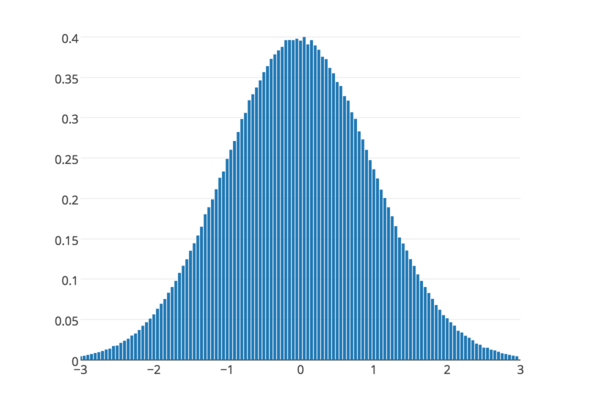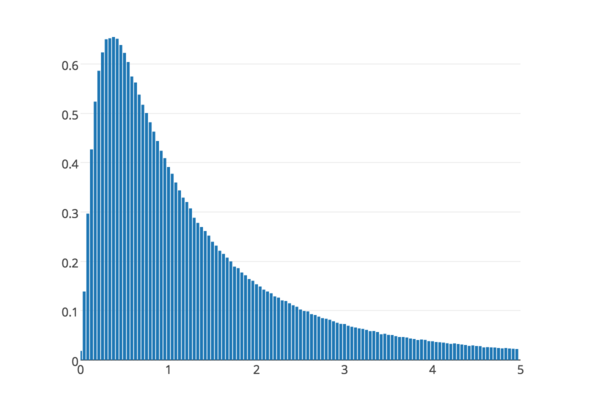# Introducing Prob.js

| random | probability | distributions | github | opensource

## Generate random numbers from different probability distributions

There are multiple libraries for generating random numbers from a uniform, and sometimes normal distributions. However, I needed code to generate them from a expontential or lognormal distribution. I had written code to do this ~10 years ago in Java, but I needed a more modern Javascript solution.

Introducing Prob.js, a javascript library to generate random numbers from different probability distributions. Avaiable via both bower and NPM, prob.js can generate random numbers from the following distrubtions:

``````Prob.uniform(min, max) // Uniform distribution in range [min, max).
Prob.normal(μ, σ)      // Normal distribution with mean and standard deviation.
Prob.exponential(λ)    // Exponential distribution with lambda.
Prob.lognormal(μ, σ)   // Log-normal distribution defined as ln(normal(μ, σ)).
Prob.poisson(λ)        // Poisson distribution returning integers >= 0.
Prob.zipf(s, N)        // Zipf's distribution returning integers in range [1, N].
After generating a distribution, the following methods are available:
``````

With a very simple to use API:

``````var r = Prob.exponential(1.0); // Create a distribution.
r()        // Generates a number within the distribution.
r(src)     // Generates a number using a `src` of random numbers. (See note below.)
r.Min      // The min random number which could be returned by `r()` (inclusive).
r.Max      // The max random number which could be returned by `r()` (exclusive).
r.Mean     // The expected mean for this distribution.
r.Variance // The expected variance for this distribution.
``````

I created a quick demo site that generates 1 million random numbers from each distribution in the browser, and plots the PDF as it goes. Same samples:Normal (μ = 0, σ = 1.0)Log-normal (μ = 0, σ = 1.0)Zipf (s = 1, N = 100)